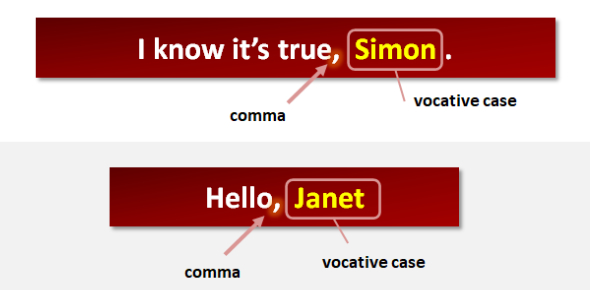# Use Cases(Grammar Quiz)

15 Questions | Attempts: 7971Settings.

• 1.
Which of the following is the most correct definition of a use case 'ACTOR' ?
• A.

An actor represents roles humans take when interacting with the system

• B.

An actor represents machines and other systems interacting with the system

• C.

An actor represents particular humans who interact with the system

• D.

An actor represents anything that interacts with the system

• 2.
What sort of UML diagram is this?
• A.

Dynamic diagram

• B.

Static diagram

• C.

Use case diagram

• D.

Use case

• 3.
Which of the following best categorizes use cases?
• A.

It is an analysis technique

• B.

It is an testing technique

• C.

It is an design technique

• D.

is is both an analysis and a design technique

• 4.
Which of the following best describes a use case ?
• A.

It is text describing in detail one flow of events through a real situation

• B.

it is the system specification problem statement

• C.

it is text which describes the dialogue between actors and the system

• D.

a diagram drawn to illustrate how cases and actors interact by sending stimuli to one another

• 5.
Use case modelling is an ________ process that enables users of a system to have input into the requirements gathering process in such a way that does not require them to have techincal knowledge and expertise outside of the role that they play in the organisation or system being modelled.What is the missing word in the sentence above?
• A.

Waterfall

• B.

Iterative

• C.

incremental

• D.

Definitive

• 6.
Why should Customers read use cases?
• A.

To approve what the system should do

• B.

To gain system understanding

• C.

as a base for test cases

• D.

As a basis for writing the user’s guide

• 7.
Which of the following outlines the structure of a typical use case in the usual order?
• A.

extends, generalizes, includes, packages

• B.

Actors, boundaries, cases, interactions

• C.

Assumptions, pre-conditions, post-conditions, flow

• D.

Description, pre-conditions, main flow, alternative flows, exceptions, post-conditions

• 8.
Why should Developers read use cases?
• A.

to gain system understanding

• B.

As a basis for writing the user’s guide

• C.

To approve what the system should do

• D.

As a base for test cases

• 9.
What is the correct name for the icon pointed to by the A in the image?
• A.

System Boundry

• B.

Actor

• C.

Relationship

• D.

Use case

• 10.
What is meant by extending a use case?
• A.

Adding exception and alternative flows to an existing base use case

• B.

Creating a new use case by adding new steps to an existing base case

• C.

Reusuing one use case's steps inside another use case

• D.

Creating a new use case by removing steps from an existing base case

• 11.
What is meant by including a use case?
• A.

Creating a new use case by adding new steps to an existing base case

• B.

creating a new use case by removing steps from an existing base case

• C.

Adding exception and alternative flows to an existing base use case

• D.

Reusuing one use case's steps inside another use case

• 12.
What is the correct name for the icon pointed to by the C in the image?
• A.

Actor

• B.

System Boundry

• C.

Relationship

• D.

Use case

• 13.
What is the correct name for the icon pointed to by the B in the image?
• A.

Actor

• B.

Use Case

• C.

System Boundry

• D.

Relationship

• 14.
What is the primary flow of a use case?
• A.

The happy day scenarios in which the way the system should work is described with the assumption that everything goes perfectly

• B.

A full description of the goals of the actor

• C.

The happy day scenarios in which the way the system should work is described with details of what happens if things go wrong

• D.

The happy day scenarios in which the way the system should work is described with all alternatives covered

• 15.
Where in a use case for Borrower Loans a Copy would the following fit? A new Loan object has been created and all data ( references to a Borrower, a Copy and the Catalog  and dates and times for the issue of the loan and when it is due) have been provided and saved and the collections of allLoans for the Catalog and the relevant Borrower have been updated.
• A.

as a pre-condition

• B.

as a post-condition

• C.

As a exception flow

• D.

As an alternative flow

## Related TopicsBack to top
×

Wait!
Here's an interesting quiz for you.